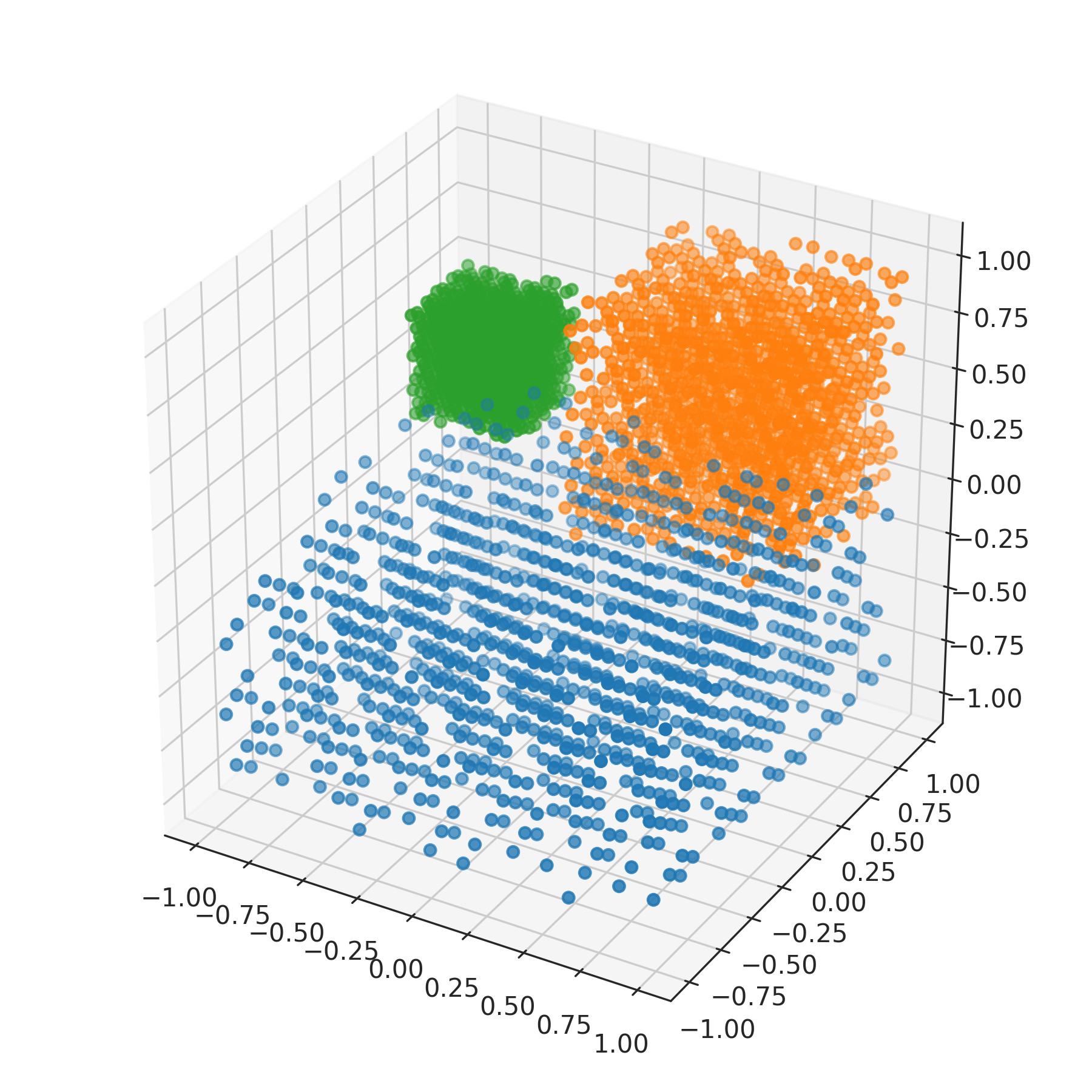nengolib.stats.ScatteredCube¶

stats.cube = ScatteredCube(low=array([-1]), high=array(), base=Rd())
class nengolib.stats.ScatteredCube(low=-1, high=1, base=Rd())[source]

Number-theoretic distribution over the hypercube.

Transforms quasi Monte Carlo samples from the unit hypercube to range between low and high. These bounds may optionally be array_like with shape matching the sample dimensionality.

Parameters: Other Parameters: low : float or array_like, optional Lower-bound(s) for each sample. Defaults to -1. high : float or array_like, optional Upper-bound(s) for each sample. Defaults to +1. base : nengo.dists.Distribution, optional The base distribution from which to draw quasi Monte Carlo samples. Defaults to Rd and should not be changed unless you have some alternative number-theoretic sequence over [0, 1].

Notes

The Rd and Sobol distributions are deterministic. Nondeterminism comes from a random d–dimensional shift (with wrap-around).

Examples

>>> from nengolib.stats import ScatteredCube
>>> s1 = ScatteredCube([-1, -1, -1], [1, 1, 0]).sample(1000, 3)
>>> s2 = ScatteredCube(0, 1).sample(1000, 3)
>>> s3 = ScatteredCube([-1, .5, 0], [-.5, 1, .5]).sample(1000, 3)
>>> import matplotlib.pyplot as plt
>>> from mpl_toolkits.mplot3d import Axes3D
>>> plt.figure(figsize=(6, 6))
>>> ax = plt.subplot(111, projection='3d')
>>> ax.scatter(*s1.T)
>>> ax.scatter(*s2.T)
>>> ax.scatter(*s3.T)
>>> plt.show()Methods

 sample(n[, d, rng]) Samples n points in d dimensions.